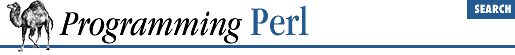#Chapter 3Functions### 3.2.167 system

`system `LIST``

This function executes any program on the system for you. It does exactly the same thing as `exec` `LIST` except that it does a fork first, and then, after the exec, it waits for the exec'd program to complete. That is (in non-UNIX terms), it runs the program for you, and returns when it's done, unlike exec, which never returns (if it succeeds). Note that argument processing varies depending on the number of arguments, as described for exec. The return value is the exit status of the program as returned by the wait(2) call. To get the actual exit value, divide by 256. (The lower 8 bits are set if the process died from a signal.) See exec.

Because system and backticks block `SIGINT` and `SIGQUIT`, killing the program they're running with one of those signals doesn't actually interrupt your program.

```@args = ("command", "arg1", "arg2");
system(@args) == 0
or die "system @args failed: \$?"```

Here's a more elaborate example of analyzing the return value from system on a UNIX system to check for all possibilities, including for signals and coredumps.

```\$rc = 0xffff & system @args;
printf "system(%s) returned %#04x: ", "@args", \$rc;
if (\$rc == 0) {
print "ran with normal exit\n";
}
elsif (\$rc == 0xff00) {
print "command failed: \$!\n";
}
elsif ((\$rc & 0xff) == 0) {
\$rc >>= 8;
print "ran with non-zero exit status \$rc\n";
}
else {
print "ran with ";
if (\$rc &   0x80) {
\$rc &= ~0x80;
print "coredump from ";
}
print "signal \$rc\n"
}
\$ok = (\$rc == 0);```